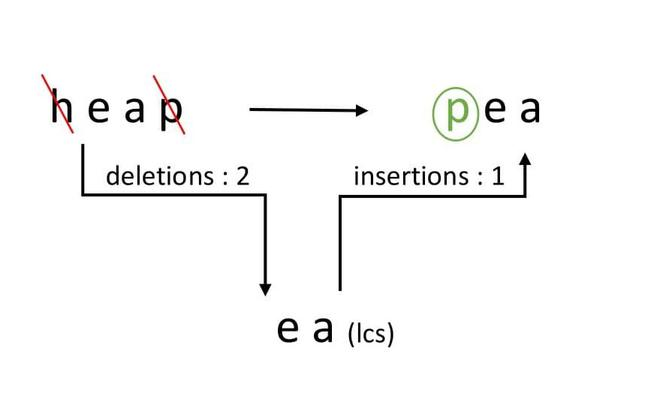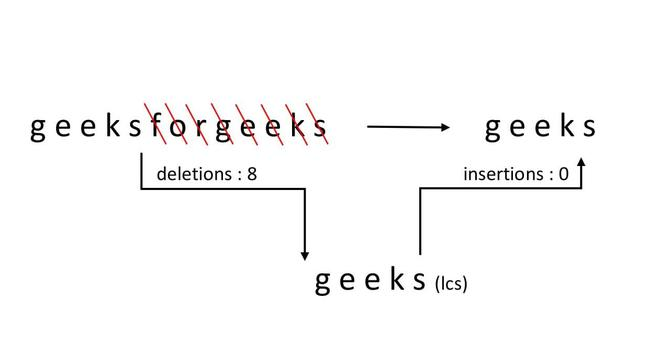Related Articles

# Minimum number of deletions and insertions to transform one string into another

• Difficulty Level : Medium
• Last Updated : 27 May, 2021

Given two strings ‘str1’ and ‘str2’ of size m and n respectively. The task is to remove/delete and insert the minimum number of characters from/in str1 to transform it into str2. It could be possible that the same character needs to be removed/deleted from one point of str1 and inserted to some another point.

Example 1:

```Input :
str1 = "heap", str2 = "pea"
Output :
Minimum Deletion = 2 and
Minimum Insertion = 1
Explanation:
p and h deleted from heap
Then, p is inserted at the beginning
One thing to note, though p was required yet
it was removed/deleted first from its position and
then it is inserted to some other position.
Thus, p contributes one to the deletion_count
and one to the insertion_count.```Example 2:

```Input :
str1 = "geeksforgeeks", str2 = "geeks"
Output :
Minimum Deletion = 8
Minimum Insertion = 0       ```Simple Approach:

A simple approach is to consider all subsequences of str1 and for each subsequence calculate minimum deletions and insertions so as to transform it into str2. A very complex method and the time complexity of this solution is exponential.

Efficient Approach:

An efficient approach uses the concept of finding the length of the longest common subsequence of the given two sequences.

Algorithm:

• str1 and str2 be the given strings.
• m and n be their lengths respectively.
• len be the length of the longest common subsequence of str1 and str2
• minimum number of deletions minDel = m – len
• minimum number of Insertions minInsert = n – len

Below is the implementation of the above code:

## C++

 `// Dynamic Programming C++ implementation to find``// minimum number of deletions and insertions``#include ` `using` `namespace` `std;` `// Returns length of length common subsequence``// for str1[0..m-1], str2[0..n-1]``int` `lcs(string str1, string str2, ``int` `m, ``int` `n)``{``    ``int` `L[m + 1][n + 1];``    ``int` `i, j;` `    ``// Following steps build L[m+1][n+1] in bottom``    ``// up fashion. Note that L[i][j] contains``    ``// length of LCS of str1[0..i-1] and str2[0..j-1]``    ``for` `(i = 0; i <= m; i++) {``        ``for` `(j = 0; j <= n; j++) {``            ``if` `(i == 0 || j == 0)``                ``L[i][j] = 0;` `            ``else` `if` `(str1.at(i - 1) == str2.at(j - 1))``                ``L[i][j] = L[i - 1][j - 1] + 1;` `            ``else``                ``L[i][j] = max(L[i - 1][j], L[i][j - 1]);``        ``}``    ``}` `    ``// L[m][n] contains length of LCS``    ``// for X[0..n-1] and Y[0..m-1]``    ``return` `L[m][n];``}` `// function to find minimum number``// of deletions and insertions``void` `printMinDelAndInsert(string str1, string str2)``{``    ``int` `m = str1.size();``    ``int` `n = str2.size();` `    ``int` `len = lcs(str1, str2, m, n);` `    ``cout << ``"Minimum number of deletions = "` `<< (m - len)``         ``<< endl;` `    ``cout << ``"Minimum number of insertions = "` `<< (n - len)``         ``<< endl;``}` `// Driver Code``int` `main()``{``    ``string str1 = ``"heap"``;``    ``string str2 = ``"pea"``;``  ` `      ``// Function Call``    ``printMinDelAndInsert(str1, str2);``    ``return` `0;``}`

## Java

 `// Dynamic Programming Java implementation``// to find minimum number of deletions and``// insertions``import` `java.io.*;` `class` `GFG {` `    ``// Returns length of length common``    ``// subsequence for str1[0..m-1],``    ``// str2[0..n-1]``    ``static` `int` `lcs(String str1, String str2, ``int` `m, ``int` `n)``    ``{``        ``int` `L[][] = ``new` `int``[m + ``1``][n + ``1``];``        ``int` `i, j;` `        ``// Following steps build L[m+1][n+1] in``        ``// bottom up fashion. Note that L[i][j]``        ``// contains length of LCS of str1[0..i-1]``        ``// and str2[0..j-1]``        ``for` `(i = ``0``; i <= m; i++) {``            ``for` `(j = ``0``; j <= n; j++) {``                ``if` `(i == ``0` `|| j == ``0``)``                    ``L[i][j] = ``0``;` `                ``else` `if` `(str1.charAt(i - ``1``)``                         ``== str2.charAt(j - ``1``))``                    ``L[i][j] = L[i - ``1``][j - ``1``] + ``1``;` `                ``else``                    ``L[i][j] = Math.max(L[i - ``1``][j],``                                       ``L[i][j - ``1``]);``            ``}``        ``}` `        ``// L[m][n] contains length of LCS``        ``// for X[0..n-1] and Y[0..m-1]``        ``return` `L[m][n];``    ``}` `    ``// function to find minimum number``    ``// of deletions and insertions``    ``static` `void` `printMinDelAndInsert(String str1,``                                     ``String str2)``    ``{``        ``int` `m = str1.length();``        ``int` `n = str2.length();` `        ``int` `len = lcs(str1, str2, m, n);` `        ``System.out.println(``"Minimum number of "``                           ``+ ``"deletions = "``);``        ``System.out.println(m - len);` `        ``System.out.println(``"Minimum number of "``                           ``+ ``"insertions = "``);``        ``System.out.println(n - len);``    ``}` `    ``// Driver code``    ``public` `static` `void` `main(String[] args)``    ``{``        ``String str1 = ``new` `String(``"heap"``);``        ``String str2 = ``new` `String(``"pea"``);``      ` `          ``// Function Call``        ``printMinDelAndInsert(str1, str2);``    ``}``}``// This code is contributed by Prerna Saini`

## Python3

 `# Dynamic Programming Python3``# implementation to find minimum``# number of deletions and insertions` `# Returns length of length``# common subsequence for``# str1[0..m-1], str2[0..n-1]`  `def` `lcs(str1, str2, m, n):` `    ``L ``=` `[[``0` `for` `i ``in` `range``(n ``+` `1``)]``         ``for` `i ``in` `range``(m ``+` `1``)]` `    ``# Following steps build L[m+1][n+1]``    ``# in bottom up fashion. Note that``    ``# L[i][j] contains length of LCS``    ``# of str1[0..i-1] and str2[0..j-1]``    ``for` `i ``in` `range``(m ``+` `1``):``        ``for` `j ``in` `range``(n ``+` `1``):``            ``if` `(i ``=``=` `0` `or` `j ``=``=` `0``):``                ``L[i][j] ``=` `0``            ``elif``(str1[i ``-` `1``] ``=``=` `str2[j ``-` `1``]):``                ``L[i][j] ``=` `L[i ``-` `1``][j ``-` `1``] ``+` `1``            ``else``:``                ``L[i][j] ``=` `max``(L[i ``-` `1``][j],``                              ``L[i][j ``-` `1``])` `    ``# L[m][n] contains length of LCS``    ``# for X[0..n-1] and Y[0..m-1]``    ``return` `L[m][n]` `# function to find minimum number``# of deletions and insertions`  `def` `printMinDelAndInsert(str1, str2):``    ``m ``=` `len``(str1)``    ``n ``=` `len``(str2)``    ``leng ``=` `lcs(str1, str2, m, n)``    ``print``(``"Minimum number of deletions = "``,``          ``m ``-` `leng, sep``=``' '``)``    ``print``(``"Minimum number of insertions = "``,``          ``n ``-` `leng, sep``=``' '``)`  `# Driver Code``str1 ``=` `"heap"``str2 ``=` `"pea"` `# Function Call``printMinDelAndInsert(str1, str2)` `# This code is contributed``# by sahilshelangia`

## C#

 `// Dynamic Programming C# implementation``// to find minimum number of deletions and``// insertions``using` `System;` `class` `GFG {` `    ``// Returns length of length common``    ``// subsequence for str1[0..m-1],``    ``// str2[0..n-1]``    ``static` `int` `lcs(``string` `str1, ``string` `str2, ``int` `m, ``int` `n)``    ``{``        ``int``[, ] L = ``new` `int``[m + 1, n + 1];``        ``int` `i, j;` `        ``// Following steps build L[m+1][n+1] in``        ``// bottom up fashion. Note that L[i][j]``        ``// contains length of LCS of str1[0..i-1]``        ``// and str2[0..j-1]``        ``for` `(i = 0; i <= m; i++) {``            ``for` `(j = 0; j <= n; j++) {``                ``if` `(i == 0 || j == 0)``                    ``L[i, j] = 0;` `                ``else` `if` `(str1[i - 1] == str2[j - 1])``                    ``L[i, j] = L[i - 1, j - 1] + 1;` `                ``else``                    ``L[i, j] = Math.Max(L[i - 1, j],``                                       ``L[i, j - 1]);``            ``}``        ``}` `        ``// L[m][n] contains length of LCS``        ``// for X[0..n-1] and Y[0..m-1]``        ``return` `L[m, n];``    ``}` `    ``// function to find minimum number``    ``// of deletions and insertions``    ``static` `void` `printMinDelAndInsert(``string` `str1,``                                     ``string` `str2)``    ``{``        ``int` `m = str1.Length;``        ``int` `n = str2.Length;` `        ``int` `len = lcs(str1, str2, m, n);` `        ``Console.Write(``"Minimum number of "``                      ``+ ``"deletions = "``);``        ``Console.WriteLine(m - len);` `        ``Console.Write(``"Minimum number of "``                      ``+ ``"insertions = "``);``        ``Console.Write(n - len);``    ``}` `    ``// Driver code``    ``public` `static` `void` `Main()``    ``{``        ``string` `str1 = ``new` `string``(``"heap"``);``        ``string` `str2 = ``new` `string``(``"pea"``);``      ` `          ``// Function Call``        ``printMinDelAndInsert(str1, str2);``    ``}``}` `// This code is contributed by nitin mittal.`

## Javascript

 ``
Output
```Minimum number of deletions = 2
Minimum number of insertions = 1```

Time Complexity: O(m * n)

This article is contributed by Ayush Jauhari. If you like GeeksforGeeks and would like to contribute, you can also write an article using write.geeksforgeeks.org or mail your article to contribute@geeksforgeeks.org. See your article appearing on the GeeksforGeeks main page and help other Geeks.